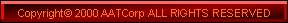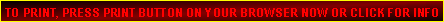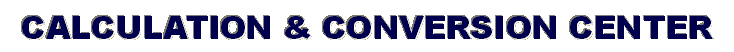GEAR RATIO CALCULATOR: Accurately determine rear & front end gear ratio with just a floor jack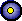BATTERY RESERVE CALCULATOR: Estimate how long a battery will last while delivering a specific load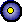CYLINDER VOLUME CALCULATOR: Determine the volume and capacity of any cylindrical object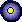AIR FILTER SIZE CALCULATOR: Figure what size air filter is required for a particular engine demand applicationCARB SIZE CALCULATOR: Estimate what size carburetor is required for a particular engine demand applicationHORSEPOWER ESTIMATE CALCULATOR: Estimate general horsepower range of an engine with minimal dataINTAKE TEMP. & HORSEPOWER CALCULATOR: Estimate power loss or gains due to air temp changesCUBIC INCH DISPLACEMENT CALCULATOR: Determine the displacement of internal combustion enginesCOMPRESSION RATIO CALCULATOR: Determine the compression ratio of internal combustion enginesENGLISH TO METRIC CONVERSION GUIDE: Convert back and forth between weights and measurements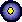CUBIC CENTIMETER TO CUBICH INCH CONVERSION: Convert displacement volumes from metric to english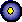LBS. PER HOUR TO GALLONS CONVERSION: Convert liquid flow into actual volumes deliveredHORSEPOWER TO TORQUE CONVERSION: Convert horsepower to torque figures at any given rpmREAR WHEEL TO FLYWHEEL HORSPOWER COVERSION: Convert rear wheel figures to estimate flywheel figuresWEIGHT TO POWER CONVERISON: Convert weight change to estimate horsepower loss or gain